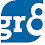# Compound Interest

Check the basics of Simple Interest HERE

The interest which is calculated NOT ONLY on the original principal, but also on the INTEREST earned in previous period is called Compound Interest.

Confused?

Ok, assume that you gave me Rs. 1000 with 10% interest. First year, the interest will be

(10/100)X1000 = 100          [Same as the Simple Interest]

But in the 2nd year, the interest will be,

(10/100) X (1000+100)  = 110

The difference between Simple Interest and Compound Interest :

• S.I and C.I are same for the first year on the same sum and at the same rate percent.
• In S.I, interest is calculated on the original Principal only (for all the years). But from 2nd year, the C.I is calculated not only on the original principal, but also on the interest of the first year.

On the same sum and at the same rate of Interest, the C.I of the 2nd year is always more than the C.I of the 1st years and their difference is equal to the interest on the interest of the first year.

 Amount = P1 + RT 100

C.I = Amt - Principal

Where,
A = Amount
P = Principal
R = Rate of Interest
T = Time Period

Example : Assume that Shivani borrows 3,000 at Rate 10% by C.I.
The C.I on any principal becomes effective only after the 1st year is complete. Which means, that at the end of the first year. So, at the first year, the C.I is equal to the S.I of the Principal

so, S.I = PTR/100  =  (3000 X 1 X 10) / 100  =  300 at the end of the first year.

NOw, if the sum borrowed with C.I, the above S.I is added to the Principal (So, in the above case, the principal becomes 3000+300 = 3300 ) and then if you calculate S.I on this amount, with the same S.I formula, you can get C.I for the second year.

P = 3300            R = 10         T = 1 Year

C.I for the 2nd year  = PTR / 100 =  (3300 X 1 X 10) / 100

C.I  = 330

The total C.I =     300 (at the end of the 1st year) + 330 (at the end of the 2nd year)

=  630

The same C.I can also be calculated by the above mentioned C.I formula.

C.I = Amt - Principal  =
Thats all for now friends. In our next post we shall discuss some problems on Compound Interest. Happy Reading :)

1.How to move to next post mam?

2.Below this comments section, you can find three options Previous, Home and Next.
By clicking on the Previous tab, you can move to the NEW posts
By clicking on the Next tab, you can move to OLD posts
By clicking on the Home tab, you will be redirected to our home page

Good Day

3.4.5.Mam pls upload sum example problems on Compound interest

6.7.hi mam,
I am not getting, how CI=amount-P
U have also written CI= P(1+R/100)-1
Can u plz explain this?

8.hi mam,
waiting since 25th Feb 2014

9.Final Amount by C.I = P(1+R/100)^T
Principal = P
Compound Interest = Final Amount- Principal
ie. P(1+R/100)^T-P = P*[(1+R/100)^T -1 ]

10.awsomstic ;-)

11.12.